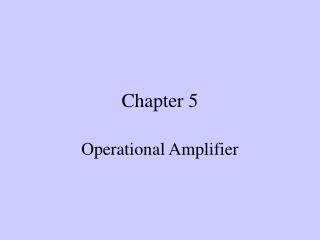# Chapter 5 - PowerPoint PPT PresentationDownload PresentationChapter 5

Download Presentation## Chapter 5

- - - - - - - - - - - - - - - - - - - - - - - - - - - E N D - - - - - - - - - - - - - - - - - - - - - - - - - - -
##### Presentation Transcript

1. Chapter 5 Operational Amplifier

2. Introduction An op amp is an active circuit element designed to perform mathematical operation of addition, subtraction, multiplication, division, differentiation, and integration. A typical op amp: a) pin configuration, b) circuit symbol

3. Terminal Voltage and Currents Vd=V2-V1 (differencial input) Vo=AVd=A(V2-V1) A: gain (open-loop voltage gain)

4. i1 i2 Actual op amp: Ri(very large); Ro(very small); A(very large) Idealization: Ri=> ; Ro=>0 ; A=>  As Vo is limited, we can get from Vo=A(V2-V1) that: V2=V1 As Ri=> , we can get i1=0, i2=0

5. i1 i2 Ideal op amp model Ideal op amp • i1=0 , i2=0 ; • V1=V2

6. Applications Inverting Amplifier

7. Applications Noninverting amplifier If Rf=0; R1=, then=>

8. + + First stage Second stage Vi Vo The voltage follower - - Applications

9. Applications Summing amplifier

10. 2 a 3 b 3 1 1 2 4 Difference Amplifier Note: be careful while using nodal analysis, do not set nodal equation at the output terminal.

11. Example: Find out Vo

12. P.P.5.10 As a voltage follower, va = v1 = 2V where va is the voltage at the right end of the 20 k resistor. As an inverter, vb = Where vb is the voltage at the right end of the 50k resistor. As a summer v0 = = [6 - 15] = 9V